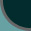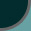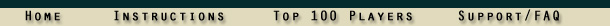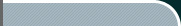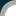Frequently Asked Questions: Dice, Probabilities, and Randomness

The dice don't seem random. What gives?
I thought computers could only generate pseudo-random numbers, not true random numbers?
I lost 10 times in a row. That's impossible with real dice! Right?
How do I calculate the probabilities?
Does that mean if I roll a die six times I'm guaranteed to roll each number once?
What's the probability of rolling at least one 6 with two dice?
That's confusing. Is there another way to look at it?
What is the probability of success in the game if I'm attacking with 1 army and the defender has 1 army?
What are the probabilities of winning other battles, such as 1 vs. 2, 2 vs. 1, 3 vs. 2, etc.?
How can you summarize those probabilities?
I was attacking a country with 2 armies from a country with 9 and I lost every single battle! How could that be?
My opponent rolled 6's five times in a row. Doesn't that seem unusual?
Something still doesn't feel right. Why do I keep seeing patterns in the rolls?
Are you absolutely certain that the random numbers used in the game are truly random?
Isn't everything just a series of causes and effects of moving particles? Is there even such thing as being random?

Q: The dice don't seem random. What gives?
A: The dice are indeed random. They are as random as throwing real dice made of plastic, wood, ivory, or any other tangible material.

Q: I thought computers could only generate pseudo-random numbers, not true random numbers?
A: There are two main ways computers can generate random numbers. When the actual variability of the numbers doesn't matter much, numbers can be generated using algorithms that derive a value from a largely unpredictable event, such as the current time measured in microseconds. These are known as pseudo-random numbers because they aren't quite as unpredictable a true random numbers. You could show someone a table of pseudo-random values next to a table of true random values, and through statistical analysis, it would be possible to determine which one was computer generated.

Because the quality of the random values matters considerably in a game like Imperial Takeover™, we don't use pseudo-random numbers. Instead, we use true random number generation. To get true randomness, we use a hardware device that collects environmental noise. The noise is digitized and then divided out into individual values. The numbers we derive are cryptographically secure true random values. You could hand someone a list of numbers generated in the game next to a list you created by rolling plastic dice, and it would be impossible to determine which was which.

Q: I lost 10 times in a row. That's impossible with real dice! Right?
A: Wrong. Very wrong. It's possible to roll nothing but 1's for the rest of your life. In every single on-line game you play, every board game, every casino game, it's possible that from now on you will roll nothing but 1's. You could roll a million dice and it's possible you'll roll a 1 every single time. It's possible, but not likely. However, losing 10 or even 20 times in a row is not only possible, but once you do the math, you'll see that it's actually not that unusual. It probably won't happen to you every day (although it could), but it will probably happen to you occasionally.

Q: How do I calculate the probabilities?
A: You can calculate the chances of a particular outcome through some fairly simple math. Let's start with a basic example. Suppose you want to know the probability of rolling one particular number, say a 4, on a single die. We start by counting up the number of total possible outcomes. We know that a die has six sides, so there are always six possible outcomes per die (1 through 6). Once we know the total number of possible outcomes, we add up the total number of desired outcomes. We know that a die is always numbered 1-6 without any duplicate values, so we know there is only one 4 on the die. Therefore, the probability of rolling a 4 is 1/6. In fact, the probability of rolling any particular number on a single die is 1/6; or 1 out of 6; or 16.67%.

Q: Does that mean if I roll a die six times I'm guaranteed to roll each number once?
A: No. The chances of a particular outcome are never affected by what has happened in the past. You could roll nothing but 1's a hundred times in a row and the chances of rolling a 1 on your 101st roll is still 1/6. Of course, it's unlikely that you would roll the same number that many times in a row.

Let's calculate exactly how unlikely it would be to roll a 1 twice in a row on a single die. We know that the probability of rolling a 1 on a single roll is 1/6. To roll a 1 two times in a row, we'd have a 1/6 chance on our first roll AND a 1/6 chance on our second roll. Because we want to know the probability of the first condition AND the second condition, we multiply: (1/6)(1/6) = 1/36, or 2.78%. What's the probability of rolling a 1 ten times in a row with a single die? Let's multiply it out: (1/6)(1/6)(1/6)(1/6)(1/6)(1/6)(1/6)(1/6)(1/6)(1/6) = 1/60466176.

So is that impossible? Not at all. The next ten times you roll a die, you could get that streak. On the other hand, maybe you'll never see that happen, but in either case, if you rolled a die ten times in a row over and over, you'd probably see that streak once every 60 million sequences. It might happen on your first sequence, it might happen on your millionth, but it will probably happen eventually.

(By the way, there's no statistical difference between rolling a single die 10 times in a row and rolling 10 dice all at the same time.)

Q: What's the probability of rolling at least one 6 with two dice?
At this point, things get a little more complicated because the easiest way to compute this is to look at the probability that something won't happen. Rolling at least one 6 on two dice is the same as looking for the probability of NOT rolling 1-5 on both dice. So we'll start by calculating the probability of not rolling a six on either dice. We have a 5/6 chance of not rolling a 6 on the first die AND a 5/6 chance of not rolling a 6 on the second die. What do we do if we want to combine probabilities? We multiply: (5/6)(5/6) = 25/36, or 69.44%. That's the chance of NOT rolling a 6 with two dice. The chance of rolling at least one six is the opposite of that: 1 - 25/36 = 11/36, or 30.56%.

Q: That's confusing. Is there another way to look at it?
A: Yes, there is. We just have to apply the same logic we always use when calculating probabilities. Count the number of desired outcomes and divide by the number of possible outcomes. Start by listing out all the possible outcomes you can get with two dice: 1,1; 1,2; 1,3; 1,4; 1,5; 1,6; 2,1; 2,2; 2,3; 2,4; 2,5; 2,6; 3,1; 3,2; 3,3; 3,4; 3,5; 3,6; 4,1; 4,2; 4,3; 4,4; 4,5; 4,6; 5,1; 5,2; 5,3; 5,4; 5,5; 5,6; 6,1; 6,2; 6,3; 6,4; 6,5; 6,6. We can see that there are 36 possible outcomes with two dice. If we want to know the probability of rolling at least one 6, we count up how many of the outcomes include at least one 6. There are 11. Therefore, the probability is 11/36.

Q: What is the probability of success in the game if I'm attacking with 1 army and the defender has 1 army?
A: If we're attacking with 1 and the defender has 1, then there are two dice involved. As we can see above, there are 36 possible outcomes with two dice. How many of those combinations would allow the attacker to win? (In the case of a tie, the defender wins.) There are 15 winning combinations for the attacker out of 36 possible outcomes. Therefore, the probability of success in a 1 vs. 1 battle is 15/36, or 41.67%. The attacker will lose 1 vs. 1 battles most of the time.

Important Reminder: We see the world in tiny slices of time. "Most of the time" applies to the universe as a whole. Most of the time, planes don't crash. If you're on a plane that's crashing, knowing that it was unlikely you'd be plunging to your death isn't going to do you a lot of good. Statistics help us predict what is likely to happen, not what's guaranteed to happen. Once something does happen, the probability that it could have happened doesn't matter a whole lot.

Q: What are the probabilities of winning other battles, such as 1 vs. 2, 2 vs. 1, 3 vs. 2, etc.?
A: With a pencil and paper it's easy enough to count up the possible outcomes, but to save you the trouble, here they are:
 3 vs. 2 2890/7776 = 37.17% (defender loses two)2611/7776 = 33.58% (each lose one)2275/7776 = 29.26% (attacker loses two) 3 vs. 1 855/1296 = 65.97% (defender loses one)441/1296 = 34.03% (attacker loses one) 2 vs. 2 295/1296 = 22.76% (defender loses two)581/1296 = 44.83% (each lose one)420/1296 = 32.41% (attacker loses two) 2 vs. 1 125/216 = 57.87% (defender loses one)91/216 = 42.13% (attacker loses one) 1 vs. 1 15/36 = 41.67% (defender loses one)21/36 = 58.33% (attacker loses one)

Q: How can you summarize those probabilities?
A: Because ties work in favor of the defender, the defender has a substantial statistical advantage. The only way to overcome that advantage is to attack with more armies than the defender is able to use. However, even a battle with 3 vs. 2 barely gives the attacker an 8% advantage.

Q: I was attacking a country with 2 armies from a country with 9 and I lost every single battle! How could that be?
A: That's certainly bad luck, but when we look at the probabilities, we see that it's not terribly unusual. Let's suppose the battle looked like this:
3 vs. 2 (attacker loses 2)
3 vs. 2 (attacker loses 2)
3 vs. 2 (attacker loses 2)
2 vs. 2 (attacker loses 2)

The probability of losing 3 vs. 2 is 29.26% and losing 2 vs. 2 is 32.41%. To compute the probability of that particular sequence, we multiply: (.2926)(.2926)(.2926)(.3241) = 0.812%. That's about 1 in 125. To put that into perspective, a typical game usually includes 500-600 rolls. It would actually be unusual not to see a string of losses like that in every single game.

Q: My opponent rolled 6's five times in a row. Doesn't that seem unusual?
A: Take a look at the following sequences and compute the probability that each one will occur:
6,6,6,6,6
4,2,1,6,3
5,5,5,5,5
2,5,3,3,1
4,4,4,4,4
1,2,3,4,5
5,1,5,2,3
Is it more likely to roll a string of 6's than a mixture of other values? No, it isn't. The probability of rolling any of those particular sequences is exactly the same: (1/6)(1/6)(1/6)(1/6)(1/6) = 1/7776

Q: Something still doesn't feel right. Why do I keep seeing patterns in the rolls?
A: The answer to this question has more to do with psychology than math. Humans have certain innate characteristics that make it extremely difficult for us to deal with randomness. We hate randomness. We hate it so much that our minds often trick us into seeing patterns where patterns don't exist and into believing that random events aren't random. We see shapes in clouds. We see faces in rock formations. When disaster strikes, we ask "why" and suspect that "something else" must be at work. The simple reality is that some things just can't be predicted. Random chance, combined with a mind always striving to see patterns can create some fascinating illusions.

Still not convinced your mind is playing tricks on you? Do some math to prove it. When rolling five dice, there are 7776 possible outcomes. How many of those would look like some sort error or pattern in the random output? All of the same number would look like a pattern. That's 6 possible outcomes right there. How about triples versus doubles, like 1,1,1,6,6 or 2,2,2,5,5? That's 25 more. How about sequential values, like 1,2,3,4,5 or 5,4,3,2,1? Once we add up all the possible outcomes that could look like something more than just random combinations of numbers, we see that there are actually quite a few. With 500-600 rolls per game, and most people playing several simultaneous games, you should see patterns all the time.

Q: Are you absolutely certain that the random numbers used in the game are truly random?
A: Yes. Without a doubt, the random numbers used on this site are every bit as random as you'd see with physical dice. We run a daily statistical analysis on our data to confirm that. Here are the results of the most recent test, which include a 40,000 point visualization, a mean computation, and a 1,000,000 value random walk test:

Q: Isn't everything just a series of causes and effects of moving particles? Is there even such thing as being random?
A: Now we're getting into the realm of philosophy. Perhaps everything that happens was all destined to occur based on the positions and velocities of particles moments after the big bang. Perhaps every star formation, asteroid collision, plane crash, thunderstorm, birth, death, and dice roll could be traced back to the way particles were bouncing around billions of years ago. Philosophers could debate these things forever, but from a practical point of view, it doesn't matter. Even if the universe operates like some sort of enormous Rube Goldberg machine, the causes and effects at work are far too complex for anyone to predict. As long as no one can predict what's going to happen, we can call it random and disregard any role "fate" might have in what comes next.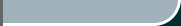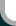Home • Bitcoin Gambling Sites • Sponsorships • Terms of Use • Privacy • Contact StrategyGameNetwork.com © 1997-2010